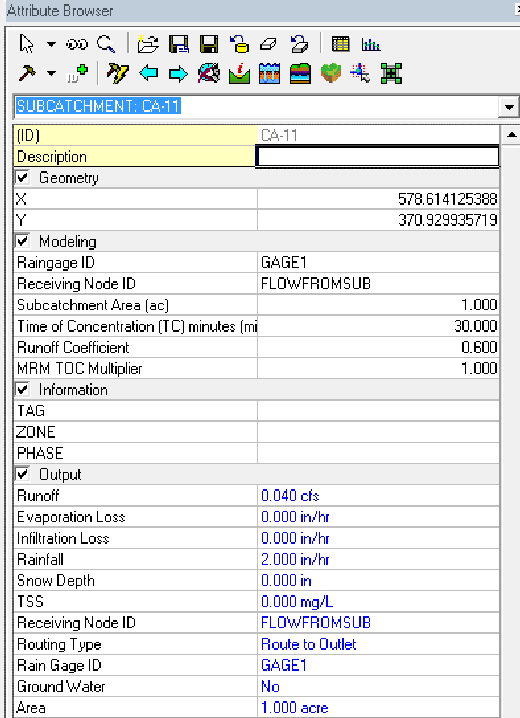## Friday, August 5, 2016

### New Modified Rational Formula in InfoSWMM

New Modified Rational Formula in InfoSWMM
InfoSWMM H2OMap SWMM now offers 12 choices for modeling surface runoff including the new Modified Rational Method (MRM)
1. EPA SWMM 5 Nonlinear Reservoir
2. The Colorado Urban Hydrograph Procedure (CUHP)
3. NRCS (SCS) Dimensionless Unit Hydrograph Method
4. NRCS (SCS) Triangular Unit Hydrograph Method
5. Delmarva Unit Hydrograph
6. Snyder Unit Hydrograph Method
7. Clark Unit Hydrograph Method
8. Espey Unit Hydrograph Method
9. Santa Barbara Urban Hydrograph Method
10. San Diego Modified Rational Method
11. Modified Rational Method (NEW!)
12. German Runoff (NEW!)
The Rational method is a widely used technique for estimation of peak flows from urban and rural drainage basins (Maidment 1993; Mays 2001). Stormwater modeling applications such as the design of detention basins require knowledge of total inflow volume obtained from runoff hydrographs. For these applications peak flow information alone may not be sufficient.  The San Diego modified rational formula is a technique adopted by the San Diego County to generate runoff hydrograph by extending the traditional rational formula. However, the San Diego Modified Rational Method (MRM) has an alternating balance hyetograph and not a constant rainfall. Starting with the release of InfoSWMM and H2Omap SWMM v13 Update 9 a new Modified Rational Method has been added with a constant Rainfall.
The peak of the runoff per Subcatchment is given by the equation Q=CIA (Figure 1).

 where, A = Subcatchment Area (hectares or acres) C = Runoff Coefficient (dimensionless and varies from 0 to 1) I = Rainfall Intensity (mm/hr or inch/hr) Q = Peak Runoff Rate (flow units)
Figure 1. Q = C I A equation definition.
The runoff option is selected as shown in Figure 2 and the Storm parameters are entered as shown in Figure’s 3 and 4
Figure 2 Modified Rational Method is the last method in the Pull Down Menu in the Runoff Model Selection Dialog. As shown in
Figure 3 The parameters for the Modified Rational Method (Runoff Coefficient, Time of Concentration and Design Storm Tc Multiplier) can be added or edited in the Attribute Browser (AB) of InfoSWMM and H2Omap SWWMM. They can also be entered using the DB Tables as shown in
Figure 4 The parameters for the Modified Rational Method (Runoff Coefficient, Time of Concentration and Design Storm Tc Multiplier) can be added or edited in the Subcatchment DB Tables of InfoSWMM and H2Omap SWWMM.
The storm parameters of the MRM are shown in Figure 6 and Figure 7
Figure 5 The Modified Rational Method has a total storm depth and either a total storm duration or a time of concentration (tc) multiplier.
Figure 6 The duration of the Modified Rational Method (MRM) is either entered as absolute hours or a time of concentration (tc) multiplier
The output of the MRM Runoff Option as shown in Figure’s 7, 8 and 9
Figure 7 The Output Manager Subcatchment Summary Table shows the peak flow, the total runoff, the time of concentration and the Simulated Runoff Coefficient.
Figure 8 The Runoff Graph can be triangular based on a constant rainfall shape if the duration is set equal to zero.
Figure 9 The Runoff Graph can be trapezoidal based on a constant rainfall shape if the duration is non zero.
Figure 2 Modified Rational Method is the last method in the Pull Down Menu in the Runoff Model Selection Dialog.Figure 3 The parameters for the Modified Rational Method (Runoff Coefficient, Time of Concentration and Design Storm Tc Multiplier) can be added or edited in the Attribute Browser (AB) of InfoSWMM and H2Omap SWWMM.
Figure 4 The parameters for the Modified Rational Method (Runoff Coefficient, Time of Concentration and Design Storm Tc Multiplier) can be added or edited in the Subcatchment DB Tables of InfoSWMM and H2Omap SWWMM.
Figure 5 The Modified Rational Method has a total storm depth and either a total storm duration or a time of concentration (Tc) multiplier.
Figure 6 The duration of the Modified Rational Method (MRM) is either entered as absolute hours or a time of concentration (Tc) multiplier
Figure 7 The Output Manager Subcatchment Summary Table shows the peak flow, the total runoff, the time of concentration and the Simulated Runoff Coefficient.
Figure 8 The Runoff Graph can be triangular based on a constant rainfall shape if the duration is set equal to zero.
Figure 9 The Runoff Graph can be trapezoidal based on a constant rainfall shape if the duration is non zero.